• opencv 图像旋转任意角度
千次阅读
2019-12-27 14:34:32
			 旋转90度
cv::Mat dst;
cv::Point center(img.cols / 2, img.rows / 2); //旋转中心
double angle = -90.0;  //角度
double scale = 1.0;  //缩放系数
cv::Mat rotMat = getRotationMatrix2D(center, angle, scale);
warpAffine(img, img, rotMat, img.size());

更多相关内容
• opencv 图像旋转（灰度级的变化）二维滤波 等
• 写了个Opencv图像缩放旋转的源码，仅供参考，免费提供
• OpenCV图像旋转首先根据旋转角度和旋转中心获取旋转矩阵，然后根据旋转矩阵进行变换，即可实现任意角度和任意中心的旋转效果。 cv2.getRotationMatrix2D(center, angle, scale) center：旋转中心 angle：旋转...

在OpenCV中图像旋转首先根据旋转角度和旋转中心获取旋转矩阵，然后根据旋转矩阵进行变换，即可实现任意角度和任意中心的旋转效果。

 cv2.getRotationMatrix2D(center, angle, scale)

• center：旋转中心
• angle：旋转角度
• scale：缩放比例

M：旋转矩阵

调用cv.warpAffine完成图像的旋转

import cv2 as cv
import matplotlib.pyplot as plt

# 1 读取图像

# 2 图像旋转
rows, cols = img.shape[:2]

# 2.1 生成旋转矩阵
M = cv.getRotationMatrix2D((cols/2, rows/2), 90, 1)  # 以图形的正中心作为旋转中心，旋转90°

# 2.2 进行旋转变换
dst1 = cv.warpAffine(img, M, (cols, rows))

M = cv.getRotationMatrix2D((cols/2, rows/2), 45, 0.5) # 旋转45°，缩小为原图的二分之一
dst2 = cv.warpAffine(img, M, (cols, rows))  # 调用warpAffine()函数完成图片旋转

# 3 图像展示
fig,axes=plt.subplots(nrows=1, ncols=3, figsize=(10, 8), dpi=100)
axes.imshow(img[:, :, ::-1])
axes.set_title("原图")
axes.imshow(dst1[:, :, ::-1])
axes.set_title("旋转后90°结果")
axes.imshow(dst2[:, :, ::-1])
axes.set_title("旋转后45°结果")
plt.show()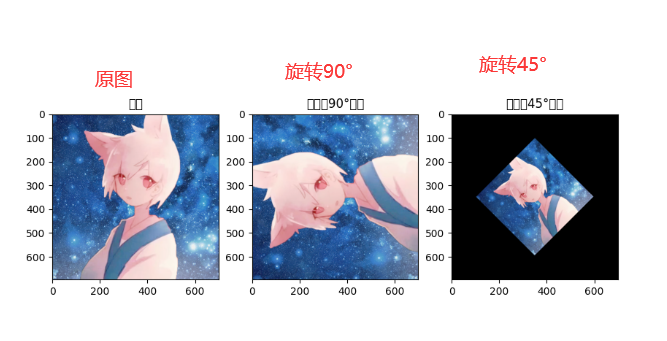展开全文• 使用opencv图像进行旋转，分为图像尺寸不变和尺寸变大两种，对尺寸变大时计算旋转后对应的坐标值。
• ## OpenCV图像旋转

万次阅读 2018-06-12 11:08:42
几何变换几何变换可以看成图像中物体（或像素）空间位置改变，或者说是像素的移动。几何运算需要空间变换和灰度级差值两个步骤的算法，像素通过变换映射到新的坐标位置，新的位置可能是在几个像素之间，即不一定为...

## 几何变换

几何变换可以看成图像中物体（或像素）空间位置改变，或者说是像素的移动。

几何运算需要空间变换和灰度级差值两个步骤的算法，像素通过变换映射到新的坐标位置，新的位置可能是在几个像素之间，即不一定为整数坐标。这时就需要灰度级差值将映射的新坐标匹配到输出像素之间。最简单的插值方法是最近邻插值，就是令输出像素的灰度值等于映射最近的位置像素，该方法可能会产生锯齿。这种方法也叫零阶插值，相应比较复杂的还有一阶和高阶插值。

插值算法感觉只要了解就可以了，图像处理中比较需要理解的还是空间变换。

## 空间变换

空间变换对应矩阵的仿射变换。一个坐标通过函数变换的新的坐标位置：所以在程序中我们可以使用一个2*3的数组结构来存储变换矩阵：以最简单的平移变换为例，平移（b1,b2）坐标可以表示为：因此，平移变换的变换矩阵及逆矩阵记为：缩放变换：将图像横坐标放大（或缩小）sx倍，纵坐标放大（或缩小）sy倍，变换矩阵及逆矩阵为：选择变换：图像绕原点逆时针旋转a角，其变换矩阵及逆矩阵（顺时针选择）为：## OpenCV中的图像变换函数

基本的放射变换函数：

[cpp]  view plain  copy
1. void cvWarpAffine(
2.     const CvArr* src,//输入图像
3.     CvArr* dst, //输出图像
4.     const CvMat* map_matrix,   //2*3的变换矩阵
5.     int flags=CV_INTER_LINEAR+CV_WARP_FILL_OUTLIERS,   //插值方法的组合
6.     CvScalar fillval=cvScalarAll(0)   //用来填充边界外的值
7. );

[cpp]  view plain  copy
2.        const CvArr* src,  //输入图像
3.        CvArr* dst,   // 提取的四边形
4.        const CvMat* map_matrix //2*3的变换矩阵
5. );
这个函数用以提取输入图像中的四边形，并通过map_matrix变换存储到dst中，与WarpAffine变换意义相同，

即对应每个点的变换：## 实践：图像旋转变换（原尺寸）

首先用cvWarpAffine实验将图像逆时针旋转degree角度。
[cpp]  view plain  copy
1. //逆时针旋转图像degree角度（原尺寸）
2. void rotateImage(IplImage* img, IplImage *img_rotate,int degree)
3. {
4.     //旋转中心为图像中心
5.     CvPoint2D32f center;
6.     center.x=float (img->width/2.0+0.5);
7.     center.y=float (img->height/2.0+0.5);
8.     //计算二维旋转的仿射变换矩阵
9.     float m;
10.     CvMat M = cvMat( 2, 3, CV_32F, m );
11.     cv2DRotationMatrix( center, degree,1, &M);
12.     //变换图像，并用黑色填充其余值
13.     cvWarpAffine(img,img_rotate, &M,CV_INTER_LINEAR+CV_WARP_FILL_OUTLIERS,cvScalarAll(0) );
14. }
逆时针旋转30度结果：这里我们将新的图像还保留原来的图像尺寸。这样的效果显然不太好，我们通过计算相应放大图像尺寸。

## 实践：图像旋转变换（保留原图内容，放大尺寸）

需要计算新图的尺寸，示意图如下：所以新图size为（width*cos(a)+height*sin(a), height*cos(a)+width*sin(a)）
[cpp]  view plain  copy
1. //旋转图像内容不变，尺寸相应变大
2. IplImage* rotateImage1(IplImage* img,int degree){
3.     double angle = degree  * CV_PI / 180.; // 弧度
4.     double a = sin(angle), b = cos(angle);
5.     int width = img->width;
6.     int height = img->height;
7.     int width_rotate= int(height * fabs(a) + width * fabs(b));
8.     int height_rotate=int(width * fabs(a) + height * fabs(b));
9.     //旋转数组map
10.     // [ m0  m1  m2 ] ===>  [ A11  A12   b1 ]
11.     // [ m3  m4  m5 ] ===>  [ A21  A22   b2 ]
12.     float map;
13.     CvMat map_matrix = cvMat(2, 3, CV_32F, map);
14.     // 旋转中心
15.     CvPoint2D32f center = cvPoint2D32f(width / 2, height / 2);
16.     cv2DRotationMatrix(center, degree, 1.0, &map_matrix);
17.     map += (width_rotate - width) / 2;
18.     map += (height_rotate - height) / 2;
19.     IplImage* img_rotate = cvCreateImage(cvSize(width_rotate, height_rotate), 8, 3);
20.     //对图像做仿射变换
21.     //CV_WARP_FILL_OUTLIERS - 填充所有输出图像的象素。
22.     //如果部分象素落在输入图像的边界外，那么它们的值设定为 fillval.
23.     //CV_WARP_INVERSE_MAP - 指定 map_matrix 是输出图像到输入图像的反变换，
24.     cvWarpAffine( img,img_rotate, &map_matrix, CV_INTER_LINEAR | CV_WARP_FILL_OUTLIERS, cvScalarAll(0));
25.     return img_rotate;
26. }

## 实践：图像旋转变换（保留原图内容，放大尺寸）-2

[cpp]  view plain  copy
1. //旋转图像内容不变，尺寸相应变大
2. IplImage* rotateImage2(IplImage* img, int degree)
3. {
4.     double angle = degree  * CV_PI / 180.;
5.     double a = sin(angle), b = cos(angle);
6.     int width=img->width, height=img->height;
7.     //旋转后的新图尺寸
8.     int width_rotate= int(height * fabs(a) + width * fabs(b));
9.     int height_rotate=int(width * fabs(a) + height * fabs(b));
10.     IplImage* img_rotate = cvCreateImage(cvSize(width_rotate, height_rotate), img->depth, img->nChannels);
11.     cvZero(img_rotate);
12.     //保证原图可以任意角度旋转的最小尺寸
13.     int tempLength = sqrt((double)width * width + (double)height *height) + 10;
14.     int tempX = (tempLength + 1) / 2 - width / 2;
15.     int tempY = (tempLength + 1) / 2 - height / 2;
16.     IplImage* temp = cvCreateImage(cvSize(tempLength, tempLength), img->depth, img->nChannels);
17.     cvZero(temp);
18.     //将原图复制到临时图像tmp中心
19.     cvSetImageROI(temp, cvRect(tempX, tempY, width, height));
20.     cvCopy(img, temp, NULL);
21.     cvResetImageROI(temp);
22.     //旋转数组map
23.     // [ m0  m1  m2 ] ===>  [ A11  A12   b1 ]
24.     // [ m3  m4  m5 ] ===>  [ A21  A22   b2 ]
25.     float m;
26.     int w = temp->width;
27.     int h = temp->height;
28.     m = b;
29.     m = a;
30.     m = -m;
31.     m = m;
32.     // 将旋转中心移至图像中间
33.     m = w * 0.5f;
34.     m = h * 0.5f;
35.     CvMat M = cvMat(2, 3, CV_32F, m);
37.     cvReleaseImage(&temp);
38.     return img_rotate;
39. }## 实践：图像放射变换（通过三点确定变换矩阵）

在OpenCV 2.3的参考手册中《opencv_tutorials》介绍了另一种确定变换矩阵的方法，通过三个点变换的几何关系映射实现变换。
变换示意图如下：即通过三个点就可以确定一个变换矩阵。（矩形变换后一定为平行四边形）
以下是基于OpenCV 2.3的代码（需至少2.0以上版本的支持）
[cpp]  view plain  copy
1. int main( )
2. {
3.     Point2f srcTri;
4.     Point2f dstTri;
5.     Mat rot_mat( 2, 3, CV_32FC1 );
6.     Mat warp_mat( 2, 3, CV_32FC1 );
7.     Mat src, warp_dst, warp_rotate_dst;
8.     //读入图像
9.     src = imread( "baboon.jpg", 1 );
10.     warp_dst = Mat::zeros( src.rows, src.cols, src.type() );
11.     // 用3个点确定A仿射变换
12.     srcTri = Point2f( 0,0 );
13.     srcTri = Point2f( src.cols - 1, 0 );
14.     srcTri = Point2f( 0, src.rows - 1 );
15.     dstTri = Point2f( src.cols*0.0, src.rows*0.33 );
16.     dstTri = Point2f( src.cols*0.85, src.rows*0.25 );
17.     dstTri = Point2f( src.cols*0.15, src.rows*0.7 );
18.     warp_mat = getAffineTransform( srcTri, dstTri );
19.     warpAffine( src, warp_dst, warp_mat, warp_dst.size() );
20.     /// 旋转矩阵
21.     Point center = Point( warp_dst.cols/2, warp_dst.rows/2 );
22.     double angle = -50.0;
23.     double scale = 0.6;
24.     rot_mat = getRotationMatrix2D( center, angle, scale );
25.     warpAffine( warp_dst, warp_rotate_dst, rot_mat, warp_dst.size() );
26.     OpenCV 1.0的形式
28.     //IplImage *img_rotate=cvCloneImage(img);
29.     //CvMat M =warp_mat;
30.     //cvWarpAffine(img,img_rotate, &M,CV_INTER_LINEAR+CV_WARP_FILL_OUTLIERS,cvScalarAll(0) );
31.     //cvShowImage("Wrap2",img_rotate);
32.
33.     namedWindow( "Source", CV_WINDOW_AUTOSIZE );
34.     imshow( "Source", src );
35.     namedWindow( "Wrap", CV_WINDOW_AUTOSIZE );
36.     imshow( "Wrap", warp_dst );
37.     namedWindow("Wrap+Rotate", CV_WINDOW_AUTOSIZE );
38.     imshow( "Wrap+Rotate", warp_rotate_dst );
39.     waitKey(0);
40.     return 0;
41. }
变换结果：写在最后的一点点闲话
别人总结出来的东西能帮助我们在一开始迅速入门，但要学深，学精，终归还是要自己去努力挖的。
展开全文• 本工程为图像旋转程序，输入图像可旋转任意角度，并输出按序号保存。旋转后的图像会被放大并且原图像未被裁剪，程序中作了对旋转后的图像缩放与裁剪，可以注释掉。工程需要配置opencv，可以使用opencv属性表，路径在...
• 点击上方“小白学视觉”，选择加"星标"或“置顶”重磅干货，第一时间送达转自|OpenCV学堂01引言初学图像处理，很多人遇到的第一关就是图像旋转图像旋转是图像几何变换中...

点击上方“小白学视觉”，选择加"星标"或“置顶

重磅干货，第一时间送达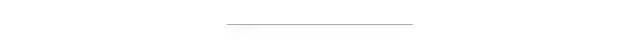转自|OpenCV学堂

01

引言

初学图像处理，很多人遇到的第一关就是图像旋转，图像旋转是图像几何变换中最具代表性的操作，包含了插值、背景处理、三角函数等相关知识，一个变换矩阵跟计算图像旋转之后的大小公式就让很多开发者最后直接调用函数了事，但是其实这个东西并没有这么难懂，可以说主要是之前别人写的公式太吓人，小编很久以前第一次接触的也是被吓晕了！所以决定从程序员可以接受的角度从新介绍一下图像旋转基本原理与OpenCV中图像旋转函数操作的基本技巧。

图像旋转基本原理

旋转涉及到两个问题，一个是图像旋转之后的大小会发生改变，会产生背景，通过背景填充方式都是填充黑色，此外旋转还是产生像素的位置迁移，新的位置像素需要通过插值计算获得，常见的插值方式有最近邻、线性插值、立方插值等。

首先看旋转之后的图像宽高变化，如下图所示：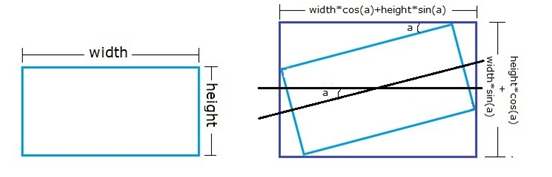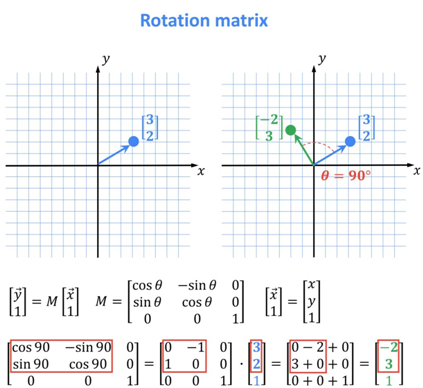这个是正常的平面坐标系中的旋转矩阵，可以简写为：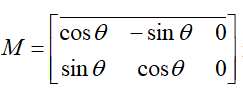是一个2x3的矩阵，但是在图像中左上角是原点，要实现围绕图像的中心位置旋转，M就要重新计算，所以OpenCV中的图像旋转矩阵为：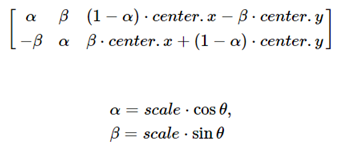其中scale是表示矩阵支持旋转+放缩，这里可以把Scale=1。第三列是图像旋转之后中心位置平移量。

函数支持

OpenCV中支持图像旋转的函数有两个，一个是直接支持旋转的函数，但是它支持的是90，180，270这样的特殊角度旋转。

void cv::rotate   (
InputArray    src,
OutputArray dst,
int rotateCode
)


其中rotateCode参数必须为：

ROTATE_180,
ROTATE_90_CLOCKWISE
ROTATE_90_COUNTERCLOCKWISE


函数warpAffine支持任意角度的旋转，通过定义M矩阵实现

void cv::warpAffine(
InputArray      src, // 输入图像
OutputArray dst, // 输出图像
InputArray      M, // 旋转矩阵
Size         dsize, // 输出图像大小
int   flags = INTER_LINEAR, // 像素插值方式
int   borderMode = BORDER_CONSTANT, // 背景填充默认为常量
const Scalar &        borderValue = Scalar() // 填充颜色默认为黑色
)


但是M如何生成与获取，OpenCV中提供了一个函数根据输入的参数自动生成旋转矩阵M，该函数为：

Mat cv::getRotationMatrix2D(
Point2f   center,
double    angle,
double    scale
)


代码演示

原图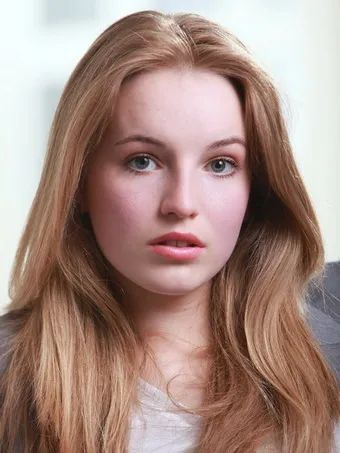使用自定义的M矩阵实现图像旋转

h, w, c = src.shape
# 定义矩阵
M = np.zeros((2, 3), dtype=np.float32)
# 定义角度
alpha = np.cos(np.pi / 4.0)
beta = np.sin(np.pi / 4.0)
print("alpha : ", alpha)
# 初始化矩阵
M[0, 0] = alpha
M[1, 1] = alpha
M[0, 1] = beta
M[1, 0] = -beta
cx = w / 2
cy = h / 2
tx = (1-alpha)*cx - beta*cy
ty = beta*cx + (1-alpha)*cy
M[0,2] = tx
M[1,2] = ty
# 执行旋转
dst = cv.warpAffine(src, M, (w, h))
cv.imshow("rotate-center-demo", dst)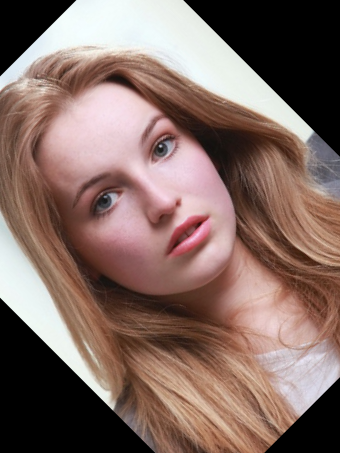重新计算旋转之后的图像大小，实现无Crop版本的图像旋转

h, w, c = src.shape
M = np.zeros((2, 3), dtype=np.float32)
alpha = np.cos(np.pi / 4.0)
beta = np.sin(np.pi / 4.0)
print("alpha : ", alpha)

# 初始旋转矩阵
M[0, 0] = alpha
M[1, 1] = alpha
M[0, 1] = beta
M[1, 0] = -beta
cx = w / 2
cy = h / 2
tx = (1-alpha)*cx - beta*cy
ty = beta*cx + (1-alpha)*cy
M[0,2] = tx
M[1,2] = ty

# change with full size
bound_w = int(h * np.abs(beta) + w * np.abs(alpha))
bound_h = int(h * np.abs(alpha) + w * np.abs(beta))

# 添加中心位置迁移
M[0, 2] += bound_w / 2 - cx
M[1, 2] += bound_h / 2 - cy
dst = cv.warpAffine(src, M, (bound_w, bound_h))
cv.imshow("rotate without cropping", dst)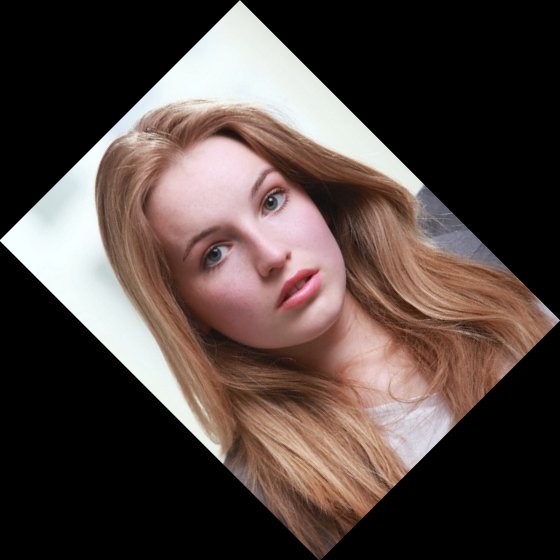背景随便变化+无Crop版本的图像旋转动态演示

degree = 1.0
d1 = np.pi / 180.0
while True:
alpha = np.cos(d1*degree)
beta = np.sin(d1*degree)
M[0, 0] = alpha
M[1, 1] = alpha
M[0, 1] = beta
M[1, 0] = -beta
cx = w / 2
cy = h / 2
tx = (1 - alpha) * cx - beta * cy
ty = beta * cx + (1 - alpha) * cy
M[0, 2] = tx
M[1, 2] = ty

# change with full size
bound_w = int(h * np.abs(beta) + w * np.abs(alpha))
bound_h = int(h * np.abs(alpha) + w * np.abs(beta))
M[0, 2] += bound_w / 2 - cx
M[1, 2] += bound_h / 2 - cy
red = np.random.randint(0, 255)
green = np.random.randint(0, 255)
blue = np.random.randint(0, 255)
dst = cv.warpAffine(src, M, (bound_w, bound_h), borderMode=cv.BORDER_CONSTANT, borderValue=(blue, green, red))
cv.imshow("rotate+background", dst)
c = cv.waitKey(1000)
if c == 27:
break
degree += 1
print("degree", degree)
if degree > 360:
degree = degree % 360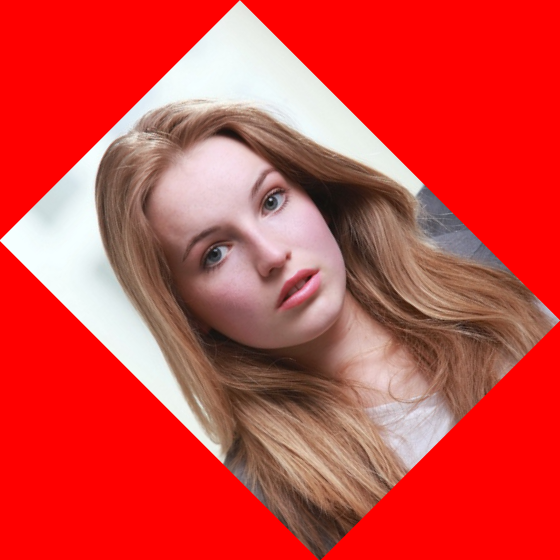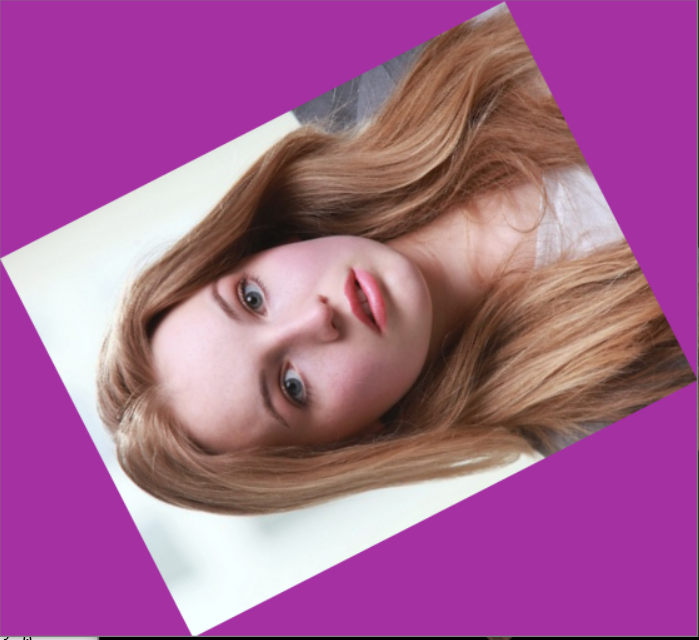下载1：OpenCV-Contrib扩展模块中文版教程

在「小白学视觉」公众号后台回复：扩展模块中文教程即可下载全网第一份OpenCV扩展模块教程中文版，涵盖扩展模块安装、SFM算法、立体视觉、目标跟踪、生物视觉、超分辨率处理等二十多章内容。

下载2：Python视觉实战项目31讲

在「小白学视觉」公众号后台回复：Python视觉实战项目31讲即可下载包括图像分割、口罩检测、车道线检测、车辆计数、添加眼线、车牌识别、字符识别、情绪检测、文本内容提取、面部识别等31个视觉实战项目，助力快速学校计算机视觉。

下载3：OpenCV实战项目20讲

在「小白学视觉」公众号后台回复：OpenCV实战项目20讲即可下载含有20个基于OpenCV实现20个实战项目，实现OpenCV学习进阶。

下载4：leetcode算法开源书

在「小白学视觉」公众号后台回复：leetcode即可下载。每题都 runtime beats 100% 的开源好书，你值得拥有！




交流群

欢迎加入公众号读者群一起和同行交流，目前有SLAM、三维视觉、传感器、自动驾驶、计算摄影、检测、分割、识别、医学影像、GAN、算法竞赛等微信群（以后会逐渐细分），请扫描下面微信号加群，备注：”昵称+学校/公司+研究方向“，例如：”张三 + 上海交大 + 视觉SLAM“。请按照格式备注，否则不予通过。添加成功后会根据研究方向邀请进入相关微信群。请勿在群内发送广告，否则会请出群，谢谢理解~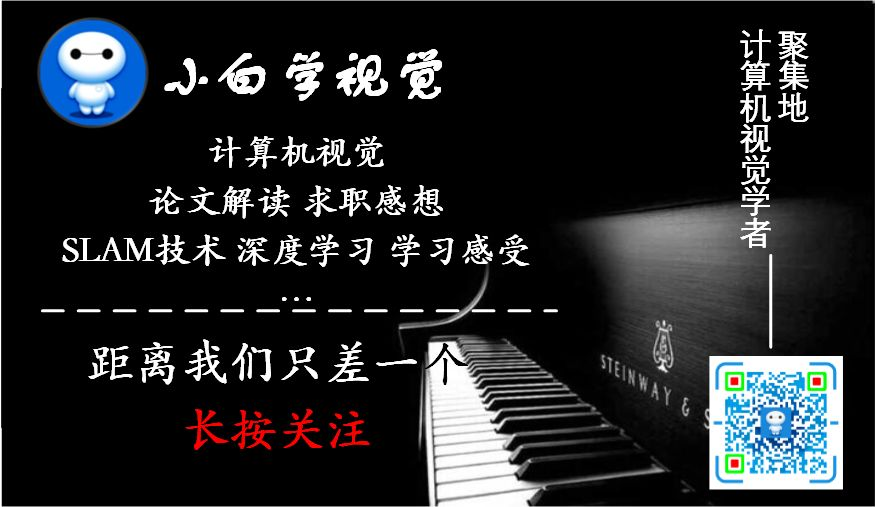展开全文python 计算机视觉 人工智能 编程语言
• 可以求出图像之间的旋转角，精度和图像大小成正比，利用频域做的，不是单纯的旋转0.1°，匹配一下，再转一下，再匹配一下。
• opencv编写实现图像旋转，用鼠标点击人脸的两个眼睛以及鼻子，即可将倾斜人脸进行校正，opencv版本为2.4.9,采用vs2012平台编写
• OpenCV是一个基于Apache2.0许可（开源）发行的跨平台计算机视觉和机器学习软件库，网络上一些小伙伴写的一些关于Android版OpenCV的博客，大部分都模糊不清，基本就复制粘贴的，有些甚至没有实践就直接贴上去了，这样...
• 主要为大家详细介绍了python opencv旋转图像，保持图像不被裁减，具有一定的参考价值，感兴趣的小伙伴们可以参考一下
• 根据txt中记录的角度旋转图像并用黑色像素补齐，通过txt中记录的斜矩形框四个点的坐标求出新坐标，对图像进行裁剪
• #include "opencv/cv.h"#include "opencv/highgui.h"#include "stdio.h"#include "iostream"using namespace cv;using namespace std;int main(){Mat src = imread("test.jpg");Mat dst;transpose(src, dst);Mat dst...
• 下面说几种OpenCV下常用的坐标变换计算，旋转、缩放、翻转和仿射变换，完全同步对应OpenCV图像处理操作。 一、旋转+缩放+翻转 代码展示的是主要逻辑，稍微改一改就可以用到项目里去。m_img是原图，m_src_corners...仿射变换 坐标变换
• 图像旋转 翻转图像 opencv中使用cv2.filp可以实现图像翻转 def flip(src, flipCode, dst=None) src：输入图像 flipCode：flipCode 一个标志来指定如何翻转数组；0表示上下翻转，正数表示左右翻转，负数表示...python 机器学习 深度学习
• opencv 2.4.9实现图像任意角度的旋转
• 文章目录参考链接：1....图像旋转90, -90, 180旋转90旋转-90旋转180 参考链接： https://blog.csdn.net/andylanzhiyong/article/details/84857915 https://blog.csdn.net/guduruyu/article/details/68...
• 点击上方“小白学视觉”，选择加"星标"或“置顶”重磅干货，第一时间送达 本文转自|OpenCV学堂01引言初学图像处理，很多人遇到的第一关就是图像旋转图像旋转是图像几何...python 计算机视觉 人工智能
• python opencv 图像旋转 原图 顺时针旋转 代码： import cv2 path = '2.jpg' img = cv2.imread(path,1) trans_img = cv2.transpose(img) new_img = cv2.flip(trans_img, 1) cv2.imshow('new_img',new_img) cv2....
•Qt
• opencv+vs2010实现图像旋转任意角度（边界正好填充），并可以实现反转回原图像大小。并做了直方图，还要有信噪比比较效果。
• OpenCV图像处理基础——基于C++实现版本视频培训课程概况：教程中会讲解到OpenCV的基础知识及使用方法，并基于OpenCV实现基础的图像处理算法；除此之外课程包含如下的内容： 图像颜色空间及类型转换及应用（BGR、YUV...计算机视觉 人工智能入门
• opencv实现图像旋转 旋转后的图像保持不变，可以更改背景颜色，利用专门的封装好的函数cvWarpAffine来实现。
• 此代码是基于VC6和opencv1.0，可以实现对任意图片任意角度的旋转。比较实用，具有参考价值。。
• 开发环境为QT5.8+opencv3.2，主要实现了图像几何变换，包括图像平移，图像旋转，图像缩放。
• ## opencv图像旋转

千次阅读 2021-11-09 16:35:16
opencv 图像旋转 double angle = rotaterect.angle; cv::Point2f center = rotaterect.center; cv::Mat M = cv::getRotationMatrix2D(center, angle, 1);//取得旋转矩阵 warpAffine(Img, Img, M, cv::Size(Img....计算机视觉...